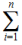Agent Adherence Reporting Metrics and End Notes

In this topic, find information about Workforce Management Agent Adherence reporting metrics and endnotes.

See Other Topics About WFM Metrics:

There is only one Adherence reporting metric.

The percentage of the day during which the agent was adherent to his or her scheduled state.

How the Percentage is Calculated

100 - ((NC+UNC)*100/(ST+UNC))

Where:

NC = Noncompliant time
UNC = Noncompliant time outside of scheduled time
ST = Scheduled time

Endnotes

This secton contains detailed descriptions and definitions for formula symbols that are used to calculate WFM metrics.

Endnote 1

The Formula for FTEs:

FTEs =(staffingi ÷ stepsHr ÷ paidHrsi x (100 ÷ (100 - pOverheadHrsi)))

Where:

n = Number of time steps in a day (96)
i = Current time step
staffing = Calculated staffing requirements
stepsHr = Time steps in one hour (4)
paidHrs = Paid Hours in a Day as specified in staffing forecast

Endnote 2

The Total/Average value of Service Level in the Contact Center Performance report is calculated as a weighted average, where for the weight coefficient we use the TotalNumberCallsDistributed configuration option.

That is, for an Activity when we define the Quality of Service Statistic type in the WFM Web, we are required to specify three statistics:

1. Service Factor
2. Distributed Interactions

(plus two time ranges required for Service Factor statistic calculation)

The Number of Distributed Interactions statistic (or TotalNumberCallsDistributed) plays the role of the weight coefficient in order to calculate the weighted average value of Service Level per:

 Timestep If we calculate Service Level for the same activity several objects (for example, across some Queues) this gives us the possibility to get an accurate result rather then a simple average. Day A weighted average gives a very accurate result in comparison with simple average.

So the calculation of Total Service Level per day is done by the formula:

SUM (SLi x TNCDi)
Service Level Total =   ————————————————————
SUM (TNCDi)

Where:

i = 1 ... 96 (intra-day 15 minute timesteps from 00:00 through 23:45)
SLi = Service Level value for the timestepi
TNCDi = TotalNumberCallsDistributed value for the timestepi

Here is a simple example of the calculation, using this data:

Timestep SL TNCD TNCDxSL
10:45 am 60.00 20 1200
11:00 am 90.00 1 90
11:15 am 20.00 150 3000
11:30 am 65.00 35 2275

If we assume that during the day we have only 4 timesteps of historical data, our Total Service Level will be:

60x20 + 90x1 + 20x150 + 65x35      6565
SL weighted = —————————————————————————————— =  —————— = 31.87
20+1+150+35                206

Here an example of calculating a simple average:

60+90+20+65
SL simple average =  ————————————— = 58.7
4

This example shows that a timestep with Service Level=90 where only one call has been processed should have minimal impact on the overall Service Level for the day, in comparison with a timestep where SL=20 and 150 calls have been processed. This is the reason the calculation is done in this way.

The user won’t be able to see TotalNumberCallsDistributed in the report, as this value is stored in the WFM database for internal purposes only (in the table WM_perf_activities in the field WM_distrib_calls, for each activity and for each timestep).

Also keep in mind that TotalNumberCallsDistributed is not the same as Interaction Volume, since in general Interaction Volume is configured as TotalNumberCallsEntered (Answered + Abandoned), while TotalNumberCallsDistributed is the number of calls which are being distributed from the queue.